Prof. Bryan Caplan

http://www.gmu.edu/departments/economics/bcaplan

Econ 345

Fall, 1998

Here are the answers for the Pre-Test. If you got less than 50% of the questions correct, you should consider dropping, because you will probably have to work very hard to get a decent grade in the class. If you got between 50-75% of the answers right, you will still find the class doable so long as you spend plenty of time on it. If you got 75-100% of the questions right, you will probably be well-prepared for this class. If you got over 75% of the questions right, and have an interest in going to graduate school, please talk to me after class or e-mail me. The graduate econometrics class I will be teaching next semester may be a better choice for you.

Find E(X), E(X2), Var(X), and SD(X), where X is:

1. A random variable generated by rolling a normal six-sided die.

E(X)=3.5 E(X2)=15.167 Var(X)=2.917 SD(X)=1.708

2. A random variable that is equal to 0 25% of the time, 1 50% of the time, 2 20% of the time, and 10 5% of the time.

E(X)=1.4 E(X2)=6.3 Var(X)=4.34 SD(X)=2.083

3. A random variable generated by doubling a single roll of a normal six-sided die.

E(X)=7 E(X2)=60.667 Var(X)=11.667 SD(X)=3.416

4. A random variable generated by summing two separate rolls of a normal six-sided die.

E(X)=7 E(X2)=54.833 Var(X)=5.833 SD(X)=2.415

Evaluate the following expressions:

1.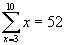2.3.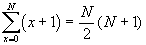4.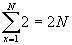Determine the Probability of the Following Events:

1. P(X-Y=0)=1/6

2. P(XY=40)=0

3. P(X2=X+Y)=2/36

4. P(X+Y<13)=1

1. P(X<5|X+Y=8)=1/3

2. P(X+Y>4|X<5)=.625

3. P(XY<10|X+Y<8)=13/18

4. P(X/Y=1|X<5)=1/4

Take the Derivative of F(x):

1. F(x)=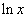; F'(x)=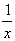2. F(x)=; F'(x)=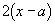3. F(x)=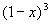; F'(x)=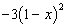4. F(x)=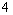; F'(x)=0